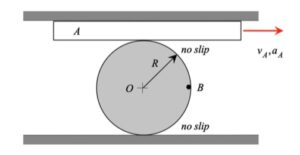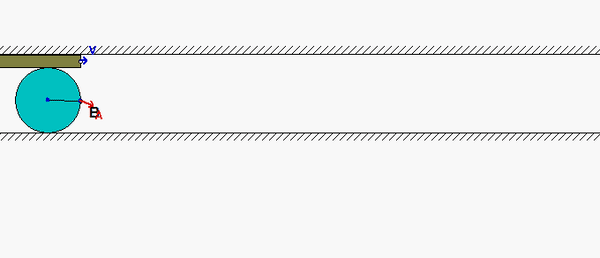# Homework H2.D - Sp23

 Problem statement Solution videoDISCUSSIONThis problem has two no-slip points on the disk (where below x is in the horizontal direction and y in the vertical direction):

• One at the bottom where the disk is in no-slip contact with the fixed ground (let's call this point C). For this point, we have vC = 0 and aCx = 0. This can be seen in the animation above in the position where B is directly BELOW O, and in no-slip contact with the ground.
• One at the top of the disk where it is in contact with the translating block A (where we will refer to this as point D). For this point, we have vD = vA i and aDx = aA. This can be seen in the animation above in the position where B is directly ABOVE O, and in no-slip contact with block A.

For this problem you will need two sets of kinematics equations:
vA = vCω x rA/C  and aA = aCα x rA/C - ω2rA/C
and:
vB = vCω x rB/C  and aB = aCα x rB/C - ω2rB/C

## 14 thoughts on “Homework H2.D - Sp23”

1.Won Jun Lee says:

Is the acceleration on point D -R(w)^2 j?

1.Sharon Ni says:

If your point D is the point between the top of circle and the surface on top of it, then your acceleration there would be alpha x r - (w)r. By looking at only the I component, since it is no slip you can set the aA (which is 3) equal to the i components to solve for alpha.

2.J Cena says:

For my equation for the acceleration of A I am getting an inconsistent solution when I break it up into i and j hat. I was wondering if a_jhat of A is equal to zero. I assumed it was because the acceleration vector for block A is only pointing in the i_hat direction. This is causing my equation to evaluate to w=0 even though the previous velocity equation I solved told me that this is false.

1.CMK says:

Yes, the acceleration of A has only an x-component. However, the point on the disk in contact with A (referred to above as "D") has both x- and y-components. The x-component of a_D is the same as a_A since it is no slip.

3.Jacob Russell Bunton says:

Is the angular velocity of point B the same as the angular velocity of the disk?

1.CMK says:

Jacob: Just to clarify, angular velocity and angular acceleration are measures of the motion of a rigid body, and are not associated with a point. That is, rigid bodies have angular velocities/accelerations, but points do not.

In short, when writing down the rigid body kinematics equations, the SAME angular velocity and angular acceleration applies for ANY two points on that rigid body.

4.J Cena says:

At the bottom of the wheel we can assume a_ihat = 0 correct?

1.CMK says:

Yes, that is what "no slip" means.

5.Christian Ladehoff says:

If you try to find a_c with respect to a_o to plug into the a_b equation provided at the top of this page, should you end up with only a j component for a_c because a_cx = 0 at a no slip point or should we keep the i component in when solving for a_b?

1.Justin James Paluch says:

I am also confused about how to find the y component of a_c which I need to solve for the acceleration of a_B.

1.Justin James Paluch says:

Nevermind.

6.Ishaan Bhargava says:

For the acceleration of point B, should there only be a i_hat direction or both a j_hat and i_hat?

1.Andrew Joseph St. Aubin says:

Ishaan:
I believe that the acceleration at B only has the i component because the question is asking for the velocity and acceleration at the position it has given you. I would assume that the velocity at point B also only has the i component as well.

1.CMK says:

No need to assume anything. Simply solve for the velocity and acceleration - math will give you the answer! (It turns out that the velocity and acceleration have both x- and y-components.)